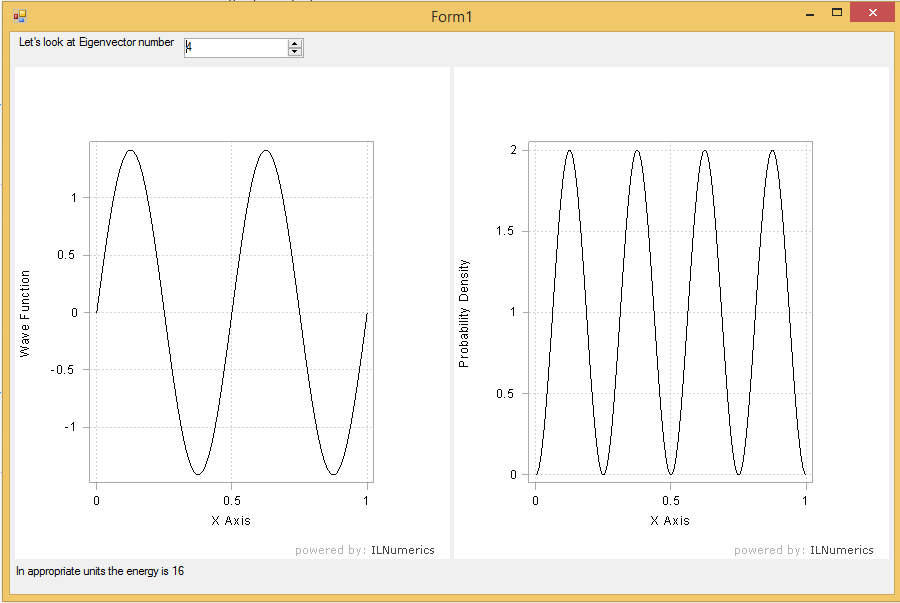# ILNumerics for Scientists – An easy start

### Motivation

I’ve been working as a scientist at universities for 10 years before deciding to go into industry. The one thing I hated most was coding. At the end of the day coding for scientists is like running for a football player. Obviously, you need it but it’s not what you’re here for.

I really dreaded the coding and the debugging. So much precious time for something that was so clear on paper and I just wanted the solution of my equations to see whether my idea made sense or not. More often than not scientists find that their idea was not so great and now they had spent so much time coding just to find out that the idea didn’t work.

To make things worse even in the case that the idea was good the code was typically in a scripted language like Python or Matlab and now needed to be rewritten in Fortran or C++. Isn’t there a better way of doing things? Isn’t there a way where the idea creation and the formulation is the bottle neck and not the coding? I could’ve spent so much more time on valuable thinking instead of senseless coding.

Now, obviously, I’m going to tell you how ILNumerics does exactly that. And, obviously, I do that because I work for them. Still, this is my experience and this is how easy it was. And at the end of the day, you’re not going to buy our product because of this blog post, rather because you downloaded our free trial and convinced yourself.

### The Problem

Let’s start with one of the simplest problems in quantum mechanics – the particle in a box. This is a quantum mechanical particle in a potential-free box with an infinite potential outside the box. In other words, the particle is forced to stay within the box. Anybody unfamiliar with this problem can read the Wikipedia article about this.

The solution to this problem is well-known and can be found analytically. As always in quantum mechanics, the solution is quantized with infinitely many solutions – also known as states. Each of the solutions consists of a wave function associated with a corresponding energy.

$\Psi_n(x) = \sqrt{\frac{2}{L}} \sin(n \cdot \pi \cdot x)$

In the equation above $n$ designates the $n^{th}$ state and $L$ is the length of the box, set to 1 in our case.

### The Goal

My goal is to display the wave function and the probability density of a user-chosen state. I choose a simple problem and a simple goal because I’m just getting started. Obviously, this is not one of the problems I faced as a scientist in my earlier life, but it is a nice example.

### The Solution

Let’s start with the mathematical part. First I implemented the wave function for a given state. As the wave function is supposed to be plotted it is discretized over a mesh. Here is my body of my function:

     ILArray<double> X = linspace<double>(0,1,MeshSize);
ILArray<double> Y = sqrt(2.0)*sin(EVID*pi*X);
return vertcat(X,Y);


The parameter EVID is the eigenvector ID, i.e. an integer greater than 0 to identify which state should be calculated. I coded the same for the density, which basically means squaring the wave function stored in vector Y.

Done in less than 10 minutes!!!

### The Graphs

The rest is all about the presentation to the user. So I created a simple Windows Form with a numeric up/down counter (to allow the user to choose the state) and two panels for simple line plots. I just needed to wire all the components to make sure that the plots were rescaled and updated after the user changes the state. This took me some more time, because it was the first time for me. Still it took me only 60 minutes and done.

### The Result

Here you can find the result of my simple little program. You can download the source code and play with it. Have fun!• Chassidy Kaspar

Timely ideas – Coincidentally , if people is wanting to merge two images , We discovered a tool here http://goo.gl/My3LN5